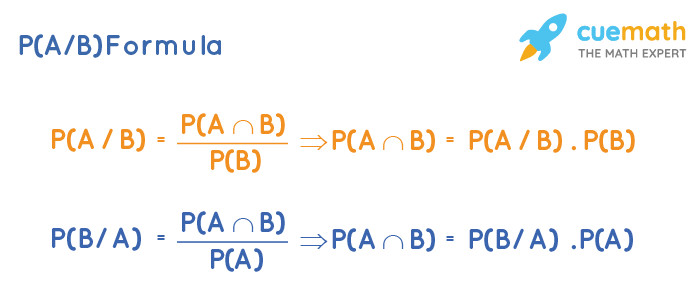# P(A/B) Formula

P(A/B) is known as conditional probability and it means the probability of event A that depends on another event B. It is also known as "the probability of A given B".  P(A/B) Formula is used to find this conditional probability quickly.

## What Is P(A/B) Formula?

The conditional probability P(A/B) arises only in the case of dependent events. It gives the conditional probability of A given that B has occurred.

### P(A/B) Formula:

P(A/B) = P(A∩B) / P(B)

Similarly, the P(B/A) formula is: P(B/A) = P(A∩B) / P(A)

Here,
P(A) = Probability of event A happening.
P(B) = Probability of event B happening.
P(A∩B) = Probability of happening of both A and B.

From these two formulas, we can derive the product formulas of probability.

• P(A∩B) = P(A/B) × P(B)
• P(A∩B) = P(B/A) ×  P(A)Note: If A and B are independent events, then P(A/B) = P(A)or P(B/A) = P(B)Have questions on basic mathematical concepts?
Become a problem-solving champ using logic, not rules. Learn the why behind math with our certified experts

Book a Free Trial Class

## Examples Using P(A/B) Formula

Example 1: When a fair die is rolled, what is the probability of A given B where A is the event of getting an odd number and B is the event of getting a number less than or equal to 3?

Solution:

To find: P(A/B) using the given information.

When a die is rolled, the sample space = {1, 2, 3, 4, 5, 6}.

A is the event of getting an odd number. So A = {1, 3, 5}.

B is the event of getting a number less than or equal to 3. So B = {1, 2, 3}.

Then A∩B = {1, 3}.

Using the P(A/B) formula:

P(A/B) = P(A∩B) / P(B)

$$P(A/B) = \dfrac{2/6}{3/6} = \dfrac 2 3$$

Answer: P(A/B) = 2 / 3.

Example 2: Two cards are drawn from a deck of 52 cards where the first card is NOT replaced before drawing the second card. What is the probability that both cards are kings?

Solution:

To find: The probability that both cards are kings.

P(card 1 is a king) = 4 / 52 (as there are 4 kings out of 52 cards).

P(card 2 is a king/card 1 is a king) = 3 / 51 (as the first king is not replaced, there is a total of 3 kings out of 51 left out cards).

By the formula of conditional probability,

P(card 1 is a king ∩ card 2 is a king)  = P(card 2 is a king/card 1 is a king) × P(card 1 is a king)

P(card 1 is a king ∩ card 2 is a king)  = 3 / 51 × 4 / 52 = 1 / 221

Answer: The required probability = 1 / 221.

## FAQs on P(A/B) Formula

### What Is P(A/B) Formula?

P(A/B) Formula is the formula used to calculate the conditional probability such that we have to find the probability of event 'A' occurring when event 'B' has occurred. P(A/B) Formula is given as, P(A/B) = P(A∩B) / P(B), where, P(A) is probability of event A happening, P(B) is the probability of event B happening and P(A∩B) is the probability of happening of both A and B.

### How to Find P(A∩B) Using P(A/B) Formula?

P(A∩B) can be calculated using the P(A/B) Formula as, P(A∩B) = P(A/B) × P(B), where, P(B) is the probability of event B happening and P(A∩B) is the probability of happening of both A and B.

### What Is ∩ Symbol in P(A∩B) Formula?

P(A/B) Formula is given as, P(A/B) = P(A∩B) / P(B), here ∩ symbol represents the intersection of event 'A' and event 'B'. P(A) is probability of event A happening, P(B) is the probability of event B happening and P(A∩B) is the probability of happening of both A and B.